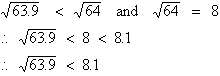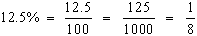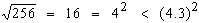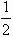Please Support the Monkey! Tell All your Friends and Teachers
 ANSWER EXPLANATIONS Drill : Section VII: Quantitative Section The values of x and y are not known and hence we cannot compare. Hence D.Hence B.Hence B.Hence B. In the 300 - 600 - 900 D ABC the side opposite 300 ( i.e. AC ) is half the hypotenuse AB i.e. AC =AB or AB = 2 AC. Hence C. In the right angled D ABC, AB2 = BC2 + CA2 and CA = CD as D ACD is an isosceles triangle. \ AB2 = BC2 + CD2 Hence C. In ( any ) D ABD, the sum of the lengths of any two sides is greater than the third side. Hence AB + AD > BD and BD = BC + CD. Hence A. PART VI Practice Tests Drill : Section 1 : Verbal Section Section 2 : Quantitative Section Section 3 : Analytical Section Section 4 : Quantitative Section Section 5 : Verbal Section Section 6 : Analytical Section Section 7 : Quantitative Section Answer Explanation To The Drill Section 1 : Verbal Section Section 2 : Quantitative Section Section 3 : Analytical Section Section 4 : Quantitative Section Section 5 : Verbal Section Section 6 : Analytical Section Section 7 : Quantitative Section